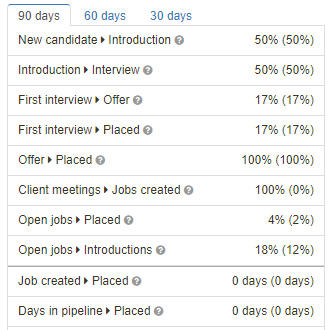The ratios widget on the dashboard shows ratios based on the past 90, 60 and 30 days. The average days numbers are calculated in overall and not within the 90/60/30 days timeframe. The number in brackets is the global number across all users in the system.New candidate > Introduction

This number shows the percentage of candidates which have an introduction with an introduced date. The date used is either the creation date, or the date this candidate has been changed into a candidate (see the dropdown on the contact record to change the type of a contact).

User : owns the candidate

Examples :

• The user created 5 new candidates, for those each candidate multiple introductions were created, each candidate has at least 1 introduction with an introduced date. This will result in a ratio of 100%
• The user created 5 new candidates, for each candidate an introduction was created, only 2 candidates have an introduction with an introduced date. This will result in a ratio of 40%
• The user created 10 new candidates and new introductions for 4 candidates, only 2 of them with an introduced date, this will show a ratio of 20%
• The user created 10 new candidates and 10 new introduction for those candidates, none of them with an introduced date, this will result in a ratio of 0%

Introduction > Interview

This number shows the percentage of introductions (an introduction with an introduced date) created within this period that has at least 1 interview. **Interview needs to be schedule through Tamago-DB by selecting "Schedule Interview" from an introduction record in the pipeline or from the pipeline grid view to correctly calculate ratios.

User : owns the job

Examples :

• 1 introduction without an interview : 0%
• 1 introduction with 1 interview : 100%
• 1 introduction with 2 interviews : 100%
• 2 introductions, only 1 has an interview : 50%

Even if an introduction has a multiple interviews, it will only count once. Introduction without an introduced date are ignored.

First interview > Offer

Shows the percentage of introductions created within this period, which have at least 1 interview, that resulted in an offer (offered date).

User : owns the job

Examples :

• The user created 20 introductions, 10 of those have at least 1 interview and 5 of those have an offered date, this will result in 50%
• The user created 10 introductions, 5 of those have an interview and none of those 5 have an offered date, this will result in 0%

First interview > Placed

Shows the percentage of introductions created within this period, which have at least 1 interview, that resulted in a placement (placed date).

User : owns the job

Examples :

• The user created 20 introductions, 10 of those have at least 1 interview and 5 of those have a placed date, this will result in 50%
• The user created 10 introductions, 5 of those have an interview and none of those 5 have a placed date, this will result in 0%

Offer -> Placed

Shows the percentage of introductions created within this period, with an offered date, that resulted in a placement (placed date).

User : owns the job

Examples :

• The user created 20 introductions, 10 of those have an offered date and 5 of those have a placed date, this will result in 50%
• The user created 10 introductions, 5 of those have an offered date and none of those 5 have a placed date, this will result in 0%

Clients met > Jobs created

Show the percentage of client companies met that resulted in created jobs. Based on the meeting and job creation dates. Meeting a company multiple times does not affect this ratio.

Examples :

• User had meetings with 5 different client companies (company is designated as being a client) this month. Out of meeting those 5 companies, jobs were created for 2 of these. Which will show as a ratio of 40%.

Open jobs > Placed

This ratio will show the percentage of job openings (a job can have more than 1 opening) versus the amount of placements. The number of placements is based on the placed date.

User : owns the job

Examples :

• The user currently has 40 active jobs which amount in a total of 50 positions. The user also placed 10 introductions within the time period. This will result in a percentage of 20%

Open jobs > Introductions

This ratio will show the percentage of job openings (a job can have more than 1 opening) versus the amount of introduction with an introduced date. The number of introductions is based on the introduced date.

User : owns the job

Examples :

• The user currently has 40 active jobs which amount in a total of 50 positions. The user created 20 introductions within the time period. Of those 20 introductions, 10 have an introduced date. This will result in a percentage of 20%

Job created > Placed

This number shows the average amount of days it takes from job creation to a first placement. Limited to the data of the past year (365 days).

User : owns the job

Days in pipeline > Placed

This number shows the average amount of days it takes from introduction created in the pipeline to its placement. Limited to the data of the past year (365 days).

User : owns the job

Introduced > First interview

This number shows the average amount of days it takes from introducing a candidate (status introduced in the pipeline) to the first interview (start time). Limited to the data of the past year (365 days).

User : owns the job